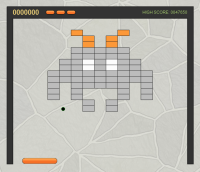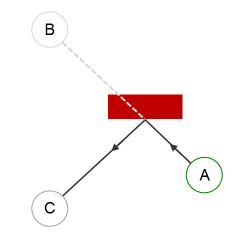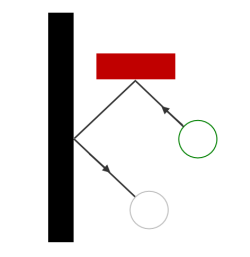# Collision Detection in Breakout

Sun, Jun 12, 2011With Breakout, collision detection becomes more complex than Pong because there are significantly more objects that the ball could collide with, and its possible, even with a small `dt` time delta, that a fast ball could collide with multiple bricks in a single frame.

## Spatial Index

Our Breakout court is, depending on the level design, up to 30 bricks wide and 25 bricks tall, meaning that we must check to see if our ball has collided with up to 750 bricks + 3 walls + 1 paddle, and we must do this 60 times a second.

You might expect that we would need the performance gain of a spatial index such as a quadtree or maybe even an r-tree, but lets start off with the simplest working solution and optimize it only when necessary.

Starting off with a simple array of bricks that we iterate through sequentially is the simplest working solution. This is an O(n) algorithm and it turns out that in our case, when `n < 1000`, all modern javascript engines can handle this just fine.

This is great, with the simplest working solution, an `update()` method that simply iterates through an array checking for a collision with each brick one at a time is fast, and is always under 1ms duration (the smallest we can measure in javascript).

So we don’t need a fancy spatial index. That will have to be an experiment that waits for another project.

## Ball & Brick Interception

The actual math of ball & brick collision is pretty much the same as we used in Pong.

And the methods we used there can be re-used for Breakout:

• `move()` - calculate new position
• `accelerate()` - calculate new position and speed
• `intercept()` - calculates the interception of 2 line segments
• `ballIntercept()` - calculates the interception of ball and rectangle

The only difference is that these methods now live in a generic Game.Math object so we can re use them across this and any future games.

You can read more about these methods in the original description for Pong

## Multiple Collisions in a Single UpdateThere is one significant difference between Pong and Breakout.

As the ball gets faster and faster it becomes more likely that the ball might collide with multiple bricks in a single frame.

Consider the case where we only have to worry about a single item (see right). If the ball is at position A at the start of the frame, we can easily calculate its position B at the end of the frame.

Next we see if the line between A and B intercepts with the surface. If it does then we simply switch the direction of the ball and place it at C, the same distance in front of the surface as it would have been behind it.But what would happen if another surface, say a wall, was close to that brick (see left)?

If we only consider the first collision within the time frame, then we would end up placing the ball behind the wall within this frame and then by the time the next frame comes around its too late, the ball has escaped magically ‘through the wall’.

What it should have done is bounced twice within a single frame (see below):Extrapolate this a little further and there can be cases, if the ball is travelling really fast, where there needs to be 3 or possibly 4 collisions within a single frame.

How do we deal with this ?

One approach is to drastically reduce the time step. Even if we are only rendering at 60fps (every 16ms), if our `update()` method is fast enough we can call it multiple times with a fixed step, say 4ms and run `update` (approx) 4 times for every `draw`.

However this relies on (a) a very fast `update` method and (b) a more complex game loop. In addition, there is still no guarantee, even with very small update time intervals, that a fast moving ball will not collide with multiple items in a single frame.

An alternative solution, and the one I used in Breakout, uses recursion to solve the problem:

• Find the closest collision
• Move the ball to the exact collision point and flip directions
• Recursively call update with the time remaining

We can find the closest collision with something like this:

``````  var n, px, brick, distance, closest = { distance: Infinity }

var p2 = Game.Math.move(this.x, this.y, this.dx, this.dy, dt);

for (n = 0 ; n < bricks.length ; n++) {
brick = bricks[n];
px = Game.Math.ballIntercept(this, brick, p2.nx, p2.ny);
if (px) {
distance = Game.Math.magnitude(px.x - this.x, px.y - this.y);
if (distance < closest.distance) {
closest = { brick: brick, point: px, distance: distance};
}
}
}
``````

If we found a closest collision point, we can place the ball at that point and switch its direction:

``````  this.setpos(closest.point.x, closest.point.y);

switch(closest.point.d) {
case 'left':
case 'right':
this.setdir(-p2.dx, p2.dy);
break;

case 'top':
case 'bottom':
this.setdir(p2.dx, -p2.dy);
break;
}
``````

Once we place the ball at the collision point, we can calculate how far along in the time interval the collision occurred and recursively call `update()` again with the time remaining:

``````  // how far along did we get before intercept ?
var udt = dt * (closest.distance / Game.Math.magnitude(p2.nx, p2.ny));

// so we can update for time remaining
return this.update(dt - udt);
``````

We recurse until no more collisions occur, and once that happens we have reached the end of our `update` frame:

``````  if ((p2.x < 0) || (p2.y < 0) || (p2.x > width) || (p2.y > height)) {
this.game.loseBall();
}
else {
this.setpos(p2.x,  p2.y);
this.setdir(p2.dx, p2.dy);
}
``````

Once I implemented a recursive update, the ball collisions became rock solid, no more phantom “through the wall” bugs - hooray!

You can find the game here and the code is here